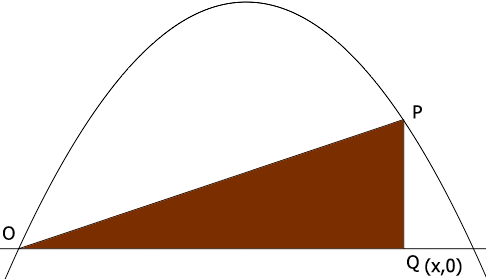SEARCH HOMEMath Central Quandaries & QueriesQuestion from bailey, a student: Hi there, A right angled triangle OPQ is drawn as shown where O is at (0,0). P is a point on the parabola y = ax – x^2 and Q is on the x-axis. Show that the maximum possible area for the triangle OPQ is (2a^3)/(27) It has been bugging me from the test. Thanks :)Hi Bailey

I expect the diagram looks something like this. I gave $Q$ the coordinates $(x,0).$What is the y-coordinate of $P?$ That's the height of the triangle. What is the area of this triangle? Use the calculus you know to find the value of $x$ that maximizes the area. What is the area at for value of $x?$

PennyMath Central is supported by the University of Regina and The Pacific Institute for the Mathematical Sciences.